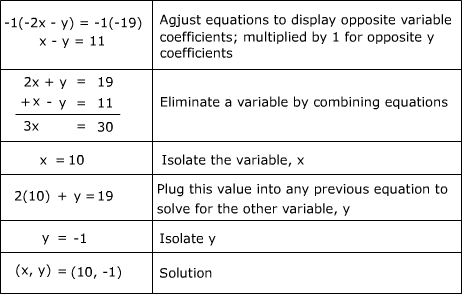# Solving Systems of Equations by Elimination : Solving Systems of Equations by Elimination Quiz

Quiz
*Theme/Title: Solving Systems of Equations by Elimination
* Description/Instructions
The elimination method is a useful tool when finding the set values to satisfy a set of equations. For the elimination method, equations are added or subtracted from each other in order to display an equation with one variable to be isolated. Once a solution to a variable is found, working backwards through equations to find other variable values is possible. Use the method of elimination to solve for(x, y) or (x, y, z) in the following systems of {e1, e2,...} equations.

Example: {-2x - y = -19, x - y = 11}Group: Algebra Algebra Quizzes
Topic: Solving Systems of Equations by Elimination